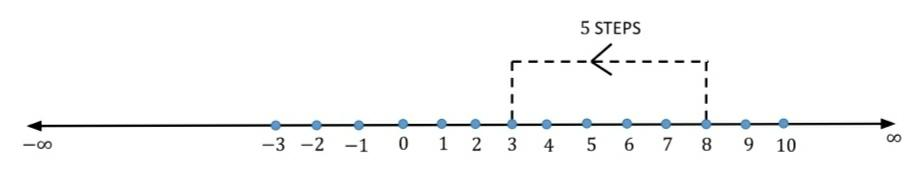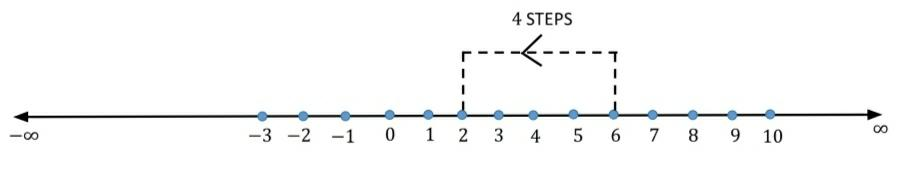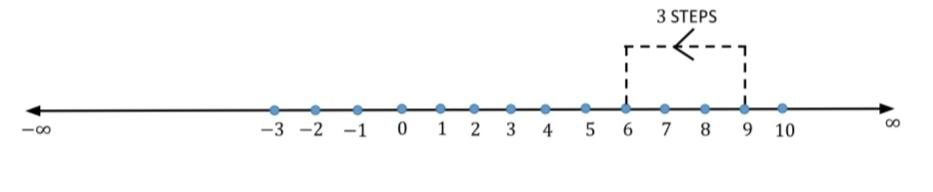QuestionAnswers

# Find $8 - 3$; $6 - 2$; $9 - 6$ using the number line.Verified
128.7k+ views
Hint: In this problem, first we will draw the number line. Then, we will mark the positions of given numbers on the same number line. Then, we will count how many steps are needed from the first number to reach the position of second number. The number of steps is the required answer.

Let us draw the number line and mark the positions of given numbers. To find $8 - 3$, we will start from the number $8$ and we will move backward to reach the number $3$.From the number line, we can say that $5$ steps are needed from the number $8$ to reach the position of number $3$. Therefore, $8 - 3 = 5$.
Now to find $6 - 2$, we will start from the number $6$ and we will move backward to reach the number $2$.From the number line, we can say that $4$steps are needed from the number $6$to reach the position of $2$. Therefore, $6 - 2 = 4$.
Now to find $9 - 6$, we will start from the number $9$ and we will move backward to reach the number $6$.From the number line, we can say that $3$ steps are needed from the number $9$ to reach the position of number $6$. Therefore, $9 - 6 = 3$.

Note:
A number line can be defined as a straight line and we can expand it infinitely in both directions. A number is usually represented by a horizontal line. To find $8 - 3$, we can start from the number $8$ and move $3$ steps backward. Similarly, to find $8 + 3$ we can start from the number $8$ and move $3$ steps forward. We move the point to the right of the number line when we need to add a positive number. We move the point to the left of the number line when we need to add a negative number.